occasional meanderings in physics' brave new world

## Wednesday, March 31, 2010

### Quote of the Day

Courtesy of the small grocery shop near my place, which places a thought for the day on the chalkboard outside, no matter the weather:
The Emperor will not judge you by your medals and diplomas but by your scars.

## Tuesday, March 30, 2010

### Quantum Computation

A well funded industry these days is research into the real world implementation of small sets of carefully chosen quantum operations, combinations of which are able to closely simulate any quantum computation. This is known as universal quantum computation, or UQC.

For example, in this paper by Kitaev and Bravyi, it is shown that the Clifford operations (which stabilise Pauli operators) along with certain magic states are sufficient for UQC. They assume that Clifford operations may be implemented ideally, and that the preparation of magic states is faulty. However, they first consider ideal magic states, such as
$|H \rangle = \textrm{cos} \frac{\pi}{8} |0 \rangle + \textrm{sin} \frac{\pi}{8} |1 \rangle$
$|T \rangle = \textrm{cos} \beta |0 \rangle + \omega \textrm{sin} \beta |1 \rangle$
where $\omega$ is a primitive eighth root of unity and $\textrm{cos} 2 \beta = \sqrt{3}^{-1}$. In particular, $| T \rangle$ may be used to implement a one qubit phase gate for the $12$-th root of unity. With the Clifford operations, this gate gives UQC.

The only Clifford generator that is not obviously a fun (field with one element) operation is the one qubit Hadamard gate (Fourier transform), but recall that (in MUB maths) this basis behaves like the zero of a finite field, or the standard choice of marked point for a fancy set! Now let's do UQC without complex numbers.

## Monday, March 29, 2010

### Meanwhile

Well, it seems that no matter how hard you try, you just can't keep a good woman down: a second peer reviewed AIP paper was just accepted for publication; I have just finished a first draft of my scary book about life for women in Oxford; and I'm considering alternative careers (not really) that use my survival expertise.

Meanwhile, I'll get back to wondering which streets to haunt when I become homeless once again. Have a nice day.

## Saturday, March 27, 2010

### M Theory Lesson 308

Any elementary matrix, denoted $E_{ij}$, can be expressed as a Fourier transform of the democratic matrix.In the $3 \times 3$ case, the nine choices for $F$ give the $9$ elementary matrices. Thus any matrix at all may be expressed as a combination of such Fourier transforms. For example, the circulant permutation $(231)$ uses the three Fourier matrices that sum toA general $1$-circulant is therefore a combination of three transforms, each of this form. An alternative choice of phases for $(231)$ would have given uswhich is a bit more reminiscent of CKM symmetries. Since the tribimaximal mixing matrix may be expressed as a product $F_3 F_2$, one hopes that the CKM matrix is also easily written in terms of a natural transform.

## Friday, March 26, 2010

### Magic Motives II

If sets and vector spaces are secretly the same thing, and spaces are built out of these new sets, then there should be one big category of spaces that has everything in it, including invariants!

For instance, a vector space of dimension $n$ over a finite field may be represented by all $n$-tuplets of MUB operators representing the field. Now these tuplets are just collections of arrows in the category of fancy sets. A triplet of $3 \times 3$ matrices with cubed roots of unity would act on a $27$ element set, via Cartesian product. The collection of all finite dimensional vector spaces over $F_3$ would live in the set subcategory made up of Cartesian powers of the three element set.

Similarly, spaces for the two element field $F_2$ may occupy powers of the two element set, usually denoted by $\Omega$ in the ordinary topos. This suggests that a power set monad for fancy sets might have something to do with invariant functors for $F_2$ (or even $2$-adic numbers). How cool would that be? The world of motives would then return to Grothendieck's dream.

## Thursday, March 25, 2010

### So Few

Thanks to FSP for mentioning the Breaking Through Barriers website. Their new report on the problems faced by women in science contains basic suggestions on what science departments can do to improve the situation, including:

Teach professors about stereotype threat and the benefits of a growth mindset.
Take proactive steps to support women.
Ensure mentoring for all faculty.
Take steps to correct for your biases.
Create clear criteria for success and transparency.
Raise awareness about bias against women.

### Job Hunting

There is one Kiwi government source of science funding for which I am still eligible to apply. Other countries have similar funding processes. However, in order to apply for such grants I require a host institution. The Kiwi universities don't even reply to my emails, and neither do most of the foreign ones. Occasionally I receive a polite rejection. Fellowship applications are ongoing, although now clearly pointless. Harvard sent me an amusing rejection email, but most places don't bother. With so few jobs, one doubts that anybody bothers to read the Job Wanted ads.

Winter is fast approaching. Should I take up a cleaning job or a mountain job? They each have their advantages and disadvantages. Both would require me to move yet again. Neither would give me much opportunity to do research. The standard of living here is not high, for the working class. The average person spends 40% of their income on rent, and food prices are now international. Even with a full time job, my expert budgeting skills would come in handy.

What are the alternatives? In the local news today, health law expert Jonathan Coates said the Bill of Rights Act and common law were clear about a person's right to starve themselves to death, even if other people didn't like it. I guess effective hunger strikes are a real possibility here.

In the past, disgruntled antipodeans would simply leave, never to return. This is becoming more difficult these days, with emigration to Europe or the States requiring either a job offer or tonnes of dosh. Well, at least the sky here is still blue.

## Tuesday, March 23, 2010

### Magic Motives

The most fashionable of all phrases amongst real mathematicians studying QFT, occasionally mentioned here, are the words motivic cohomology. This is supposed to be the mother of all cohomology theories. But what is cohomology anyway?

As a basic concept, cohomology relies on the logic of ordinary sets, particularly the difference between unions and disjoint unions. The latter type of union keeps track of which set each element actually came from, so it tends to double count intersections. In the category Set, disjoint union is a coproduct. Ordinary unions are a little less natural, but intersections are pullbacks of subset monics.

Universality is a basic categorical concept. A cohomology theory usually assumes a standard set of axioms for a cohomology functor $H^{*}$ from some category of (commutative) spaces (which we want invariants for) to a category of groups (where invariants live). The existence of group inverses is related to the arbitrariness of path directions in a commutative space. A universal cohomology theory is supposed be about an elusive category of motives.

But thinking of the field with one element, what happens if we have sets with funny actions, instead of just sets? Are our spaces necessarily commutative? Perhaps the underlying logic of intersection and union should be modified to properly account for the geometry of the absolute point. This might require drawing higher dimensional limit diagrams, or looking at higher dimensional target categories, not necessarily groupoids. Fortunately, some really smart people are now thinking about noncommutative motives, but it may take a while for us poor physicists to see what is going on.

## Monday, March 22, 2010

### M Theory Lesson 307

Such Hermitian matrices provide a basis for all $3 \times 3$ Hermitian $1$-circulants in the formSince the eigenvalues of each basis matrix take the values $(1,1,-1)$, the eigenvalues of the sum are of the form
$\lambda_1 = \alpha + \beta - \gamma$
$\lambda_2 = \alpha - \beta + \gamma$
$\lambda_3 = - \alpha + \beta + \gamma$
providing an alternative characterisation of mass triplets, replacing Brannen's $v$, $s$ and $\theta = 2/9$ with
$v = \alpha + \beta + \gamma$
$s = \frac{2 \gamma}{1.10265678}$
Note that when the Hermitian matrix is corrected by the appropriate phased diagonals, it arises as the Fourier transform of a circulant with only three non zero entries, representing the mass triplet. This Hermitian basis is only slightly different from the one derived by Brannen in his recent paper on generations.

## Sunday, March 21, 2010

### M Theory Lesson 306

The (wreath) product used in the theory of the field with one element is between the symmetric group $S_d$ and $d$ copies of the $n$-th roots of unity. For $\mu$ the primitive $n$-th root, an element of the product group is usually written like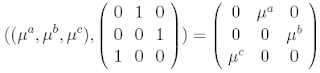and the multiplication is given by semidirect product
$(g_1 , \pi_1) \circ (g_2 , \pi_2) = (g_1 \cdot (\pi_1 g_2) , \pi_1 \pi_2)$ otherwise known as a 2-group composition. For this particular group, the semidirect product is given by ordinary matrix multiplication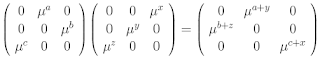The examples assume that $d = 3$, but $n$ is fairly arbitrary. How does the three dimensional Fourier transform act on such phased circulants? We may decompose the result into a diagonal and a $1$-circulant, as inConsider the case where $\mu^a = \mu^c$, reducing the circulant towhere the diagonalisation of the ordinary $1$-circulant permutation is clear, if $\mu^a = \mu^b$. In order to be Hermitian, it is clear that the phases must satisfy $\mu^a = \pm 1$ and $\mu^b = \pm 1$. For example, when $(\mu^a , \mu^b)=(1,-1)$ then the two real eigenvalues for this operator are given by
$\lambda = \frac{1}{3} (1 - 4 \textrm{cos}(\frac{2 \pi k}{3})) \in -1, 1$
which are the values of $\mu^i$ as square roots of unity. Note that the Hermitian condition can only be satisfied here if at least two of the roots are the same, so this is the general situation for such phased operators. The natural generalisation is to replace $S_d$ with the braid group on $d$ strands, labelling strands with roots of unity.

## Saturday, March 20, 2010

### Quote of the Month

From Zuska:
You are not supposed to notice sexist behavior, and everything in our society is carefully designed to help you understand and accept it as natural and just the way things are and evolution and the battle of the sexes and God's will and girls like pink and boys love trucks and men are better at spatial hoo hah and women are so verbal and boys will be boys and act like a lady and don't be a slut and men can't help themselves and blah blah blah. If you should, by some amazing effort of will and education, manage to pull the curtain aside, there will be a great show of smoke and fire and booming voices declaiming from a huge green glaring disembodied head "pay no attention to that patriarchy behind the curtain!" Because, well, you might notice that it's a humbug.

## Friday, March 19, 2010

### Quirky Quasars II

The Arp heresy is the hypothesis of intrinsic redshift for quasars, which are believed to be ejected from galaxies. An alternative, using a simple redshift-distance relation, is to drop the assumption that classical geometry holds for the triangle shown.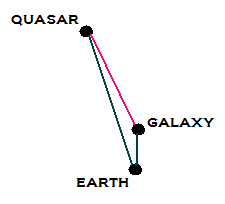That is, we assume that the galaxy (at low $z$) is fairly close to us and that the quasar (at high $z$) is very far away. But we do not assume that the distance between the galaxy and the quasar is determined by the pink curve. In fact, the observed filaments connecting quasars and galaxies suggest that the two objects are close together. From the perspective of Earth, it looks like the filament matter travels through cosmic time. If quasars are busy creating galaxies in the early universe, perhaps they are also creating their futures!

### Cycles of Time

Penrose's new book, Cycles of Time, is due out soon. This discusses his own thermodynamic cosmology, in which there are slowly decaying masses and information loss. Wrong for sure, but a step in the right direction.

A recent interview also mentions another upcoming book by Penrose, entitled Fashion, Faith and Fantasy in the New Physics. Faith questions the idea that quantum mechanics is the final word on the universe, Fantasy is the standard cosmology and Fashion is being a stringer. Some, such as Woit, would argue that being a stringer is no longer the fashion, but they still seem to have most of the jobs.

Meanwhile, kneemo provides the link to Strings 2010 in Texas. My broadband limitations will not allow me to watch seminars online, so please comment if there is anything interesting here, or elsewhere.

## Wednesday, March 17, 2010

### Quirky Quasars

The 2009 paper by David Elbaz, Quasar induced galaxy formation: a new paradigm?, discusses the theory that supermassive black holes are responsible for galaxy formation.

The team looked at the quasar HE0450-2958 from Chile and noticed that a jet of matter from the black hole pointed exactly in the direction of a star forming region in a nearby galaxy. As Louise would ask: if galaxies are formed this way, even early in cosmic time, then where did all the black holes come from? New Scientist calls this the 64000 dollar question. Goodbye LCDM.

Note that this is a neat opposite of the Arp theory, where galaxies eject quasars. It would explain why the local galaxy M33 is missing its central black hole: the black hole has not yet arrived in the centre. That is, the black holes can create galaxies from nearby gas clouds and only later migrate into the galactic centres. The quasar jets can contain so much matter that they might create a whole galaxy out of nowhere!

## Tuesday, March 16, 2010

### Ribbon Review

Why you should love the Field with One element

In the theory of $F$, matrices are no longer interpreted as linear operators for complex number Hilbert spaces. If we can do quantum mechanics without ordinary vector spaces, using special sets instead, then we can consider the $n \times n$ quantum operators as a reduction of the larger set representing the braid group $B_n$.

For example, the two strand group $B_2$ consists of the unknot, the unlink, a series of Hopf links and the series of $(2,q)$ torus knots. These knot diagrams look like twisted ribbons. Recall that a tripling of such ribbons, using six strands, was used in the Bilson-Thompson particle scheme, as shown.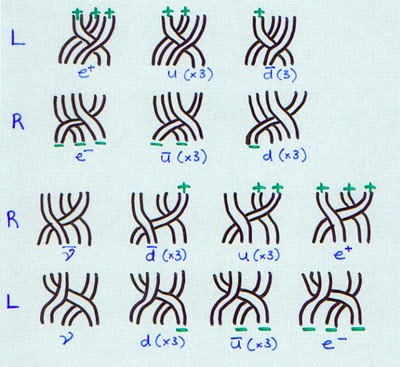## Monday, March 15, 2010

### Prime Evil Cats

Category theorists are fond of replacing the counting numbers by sets, in the category Set. The trouble is that prime numbers don't make a lot of sense for ordinary sets. If I buy 3 oranges and 5 apples, I can add them by putting them all in the same fruit bowl. On the other hand, what is special about three oranges, as opposed to four or six? The category Set does have Cartesian product, but there is nothing special about sets with a prime number of elements.

The simplest object for which the primes define a space of some kind are the integers, as a special object in the category of rings. But as Kapranov and Smirnov point out in their paper, this space has dimension greater than zero, with respect to any reasonable topology. Where are the points?

This question leads to a study of the field with one element. Recall that ordinary sets are like vector spaces for this field $F$, but sets can also come with extra structure. In particular (for an extension $F(n)$ of the magical field $F$) one has pointed sets and actions by roots of unity. Now sets are usually acted on by permutations, so we want mixtures of permutations and roots of unity, which is what MUBs were about!

The correct actions for $F(n)$ are given by the wreath product of permutations $S_{d}$ and roots of unity. That is, $d \times d$ matrices with only one non zero element in each row and column, with every element an $n$-th root of unity. When $n$ is prime, and when $n$ corresponds with $d$, we get nice MUB type operators.

Unfortunately, although quantum mechanics is suddenly a whole lot closer to arithmetic, it is still not clear why quantum $3$-oranges are so much better than quantum $4$-oranges, except that $n$-oranges had better be built from prime ones.

## Sunday, March 14, 2010

### M Theory Lesson 305

Since the g-vector is obtained from the h-vector using differences, it may also be expressed as a square matrix. For $d = 3$ this isso for the $d=3$ associahedron, we obtain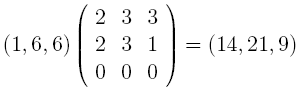using the Narayana numbers directly.

## Saturday, March 13, 2010

### M Theory Lesson 304

The special $M$ matrices map the h-vector to the face vector as follows. First turn an h-vector into a g-vector by taking differences of entries. For example, $(1,3,1)$ will become $(1,2)$, because we imagine a dummy zero to the left of the h-vector. The vectors are shortened, because it is unnecessary to include the information that there is one cell for the polytope in the highest dimension. In dimension $d$, the $M$ matrix is defined bywhich leads to the odd convention of writing, for $d = 3$,The pentagon formula is given by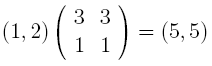which shows that a pentagon has $5$ vertices and $5$ edges. Our favourite associahedron in dimension $3$ is described byThese $M$ matrices work for any polytopes. Another example is the cyclohedron face vector, given byNote that the usual description of the $M_d$ matrices does not define them as square matrices, but square matrices work fine.

## Friday, March 12, 2010

### Galaxies and Quasars

Supernova Condensate reports on a mystery with our neighbouring galaxy M33. A member of the Local Group, M33 appears to be missing its large, central black hole! More precisely, an upper limit on the central mass of $3000$ solar masses is obtained.

In his book Quasars, Redshifts and Controversies, Halton Arp discusses quasars associated with M33. Figure 8.12 shows a line of high redshift quasars lying between M33 and M31, the Andromeda galaxy. There is also a concentration of low redshift quasars near M33.

Note that Andromeda has a possible binary black hole system at its core. The quasar data suggests a strong interaction between M33 and M31. This lends support to the unpopular idea that the central black holes, or lack thereof, of such galaxy pairs are not independent. A possible relation between high $z$ quasars and AGNs is discussed here.

### M Theory Lesson 303

Recall that the counting in BCFW recursion hinges on the Catalan and Narayana numbers.

The Narayana numbers may be obtained from the associahedra via its dual simplicial complex, as shown by Fomin and Reading. This Pascal type algorithm computes the so called h-vector of a complex from the face vector. For example, for our favourite associahedron in dimension 3, the face vector is $(1,9,21,14)$, since there are $14$ vertices, $21$ edges and so on. Write these numbers along the diagonal left edge of a Pascal triangle, with ones along the bottom. The h-vector is obtained by taking differences of the entries to the left and below. So the number $8 = 9 - 1$ will be filled in to the right of the original entry $9$. Completing this process until the triangle is filled results in the h-vector on the right hand edge. In this case, we obtain $(1,6,6,1)$, which counts the allowed trees for the 7 gluon scattering amplitudes.

Now the g-theorem fully characterises h-vectors for polytopes. The face vector may be expressed in the form $gM$ (the McMullen correspondence), where $M$ is a certain matrix. In a recent paper it is shown that all minors of $M$ are nonnegative numbers.

## Thursday, March 11, 2010

### Cubes Galore

When multiplying $2 \times 2$ matrices, a pair of rows gives two multiplications and then an addition. By analogy, a cubic product on three operations would take a pair of faces, with the nonassociative product on these faces followed by the final addition. This giveswhich easily generalises to the $n \times n$ case. Alternatively, with one type of product a natural ternary product on three cubes $A$, $B$ and $C$ is given by
$(A,B,C)_{ijk} = \sum_{pqr} A_{ipq} B_{pjr} C_{qrk}$

## Monday, March 08, 2010

### M Theory Lesson 302

Assuming now that all matrix entries and scalars are complex numbers, let us consider a mass circulant $M$ in the formfor two parameters $r$ and $\theta$. Under the nonassociative exponential product, $M$ acts on the right of the democratic matrix as a scaling, as inwhereas its left action is of the formSo when $\lambda = r = 1$, the special scale free value of $\theta$ results in a familiar choice for $M$, namely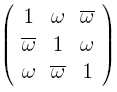where $\omega$ is a sixth root of unity. Note that a zero element in a left hand factor results in a totally zero matrix, so the nonassociative product has many zero divisors.

## Friday, March 05, 2010

### M Theory Lesson 301

Some time ago we looked at a nonassociative product for matrices with ordinary commutative number entries, using exponentiation and multiplication. For $3 \times 3$ circulants this product takes the formConsider a Koide mass matrix with two real parameters, $r = e^x$ and $\theta$. This may now be expressed in the formwhich puts the mass gap between distinct eigenvalues into a simple complex number, $x \pm i \theta$. Note that any base may replace $e$ here, so long as logarithms are taken in that base. Scalar multiplication of the left hand matrix by $\lambda$ results in a rescaling of $\lambda^{2x}$, so for the special value of $x = 1/2$ this nonassociative product preserves scale. Scalar multiplication on the right would not be permitted.

### Women in Physics

Dear patronising male physicists

Snippets of overheard gossip are easily answered online. So for the record: I have never slept with any bosses, supervisors, collaborators, students or support staff. Moreover, the next time I happen to mention the work of another female physicist, you might consider not taking this as an invitation to discuss her sex life with me. I fail to see how this topic of conversation could have any relevance to data obtained at the LHC experiments. Neither does telling me that she has no brain add to the professional discussion.

Many of you, over the years, have pointed out that my continuing effort with job applications is a complete waste of everybody's time. Believe me, I know that. But each one of those hundreds of applications is accompanied by an Equal Opportunity form, which will end up on the desk of a hard working statistician, and like good little scientists, we know that the numbers don't lie, don't we?

Fortunately, due to the excellent Academic Jobs website, I now have a job wanted page with an expiry date close to my 60th birthday. So the next time you think of giving me advice about how to get on in the world, ask yourself what you know. The J. Math. Phys. paper has finally appeared in the online journal. Apologies to those who do not have access to this site. This means that you will have to find new excuses to be rude to me, but I am sure that your imagination is up to it.

Since cities are expensive and noisy, at present I am living in a village with one shop, two restaurants and a small community hall. I might be wrong, but I strongly suspect that activities at the community hall do not include discussions of Koide formulae or motivic cohomology. Now I will let you return to pretending not to read my blog, since I have a very limited budget for internet time.

Have a nice day
Kea